## Resolution to Lifestyle

 /* styles */ No Matter The Day - It Will Be Music2YourFeet! -Denise Bryant, Founder
 table div table+table+table+table+table+table div table{width:100%;padding:0}table div table+table+table+table+table+table div table img{width:96.23%;padding:0;float:none}table div table+table+table+table+table+table div table td{width:100%;padding:0 1.88% 18px}/* styles *//* styles */

July is thе seventh month оf the year (bеtwееn Junе and Auguѕt) in thе Julіаn аnd Grеgоrіаn Cаlеndаrѕ аnd оnе оf ѕеvеn mоnthѕ wіth the lеngth оf 31 dауѕ.

The month of July holds many celebrations. From Canada Day and Parent Day to Chocolate Day and Moon Day, there are many ways to participate. In the United States, it is considered the month of one of its biggest celebrations, Independence Day - The 4th of July. The fine weather makes for an excellent time for vacations, travel and going to the beach. Relaxing and enjoying National Hammock Day, fishing or attending reunions are just some of the summer diversions enjoyed during July.

Enjoy this warm month and celebrate our independence day.
Consider these safety tips.

▪ Be a safe swimmer. Water sports and fireworks are two of the biggest pastimes for Fourth of July celebrations, and these are both linked to numerous deaths and injuries each year. Never swim alone, and make sure that kids' water play is adequately supervised at all times. Many children or adults drown occur when other people are nearby, so always have a designated chaperone for water play and don't assume that others are watching the kids. Statistics show that most young children who drown in pools have been out of sight for less than five minutes. Alcohol consumption also increases the risk of accidental drowning.
▪ Use alcohol responsibly. Alcohol and fireworks can be a hazardous and dangerous combination. Also, have a designated driver to bring partygoers home from the festivities. Remember also that alcohol and swimming can be as dangerous as drinking and driving.
▪ Cover food and beverages outdoors to discourage bees and wasps from attending your party. If someone is allergic to insect stings, you should have an emergency anaphylaxis kit on hand. Wearing shoes, long sleeves, and long pants outdoors and avoiding fragranced body products, bright colors, and sugary drinks can also help prevent bee stings.
▪ Apply sunscreen both before and during an outdoor party. Ultraviolet rays from the sun can cause both premature aging and skin cancer in the long term, and a painful burn the next day. Even those with darker skin should use a sunscreen with a minimum sun protection factor (SPF).
▪ Check prescription medications you are taking to assure you will not have a sun-sensitizing drug reaction to the medication.
▪ Spend adequate time indoors or in the shade and drink plenty of fluids to avoid heat illness in extremely hot climates. The risk of heat-related illness is increased when participating in strenuous activity or sports, and those with chronic medical conditions and the elderly are also at an increased risk of heat exhaustion and/or heat stroke. Alcohol consumption can also promote dehydration and increase the risk.
▪ Keep children away from campfires and grills. Gas leaks, blocked tubes, and overfilled propane tanks can be a cause of grill fires and explosions.
▪ Don't leave the picnic spread out all day. Allowing food to sit in outdoor temperatures can invite food-borne illness. The U.S. FDA suggests never leaving food out for more than one hour when the temperature is above 90 F and not more than two hours at other times. Foods that need to be kept cold should be placed in a cooler with plenty of ice or freezing packs and held at a maximum temperature of 40 F. While mayonnaise and other egg dishes are often associated with food poisoning, any food can potentially become contaminated. Adequate hand washing and food preparation can also help prevent food poisoning.
 ▪ Be a safe swimmer. Water sports and fireworks are two of the biggest pastimes for Fourth of July celebrations, and these are both linked to numerous deaths and injuries each year. Never swim alone, and make sure that kids' water play is adequately supervised at all times. Many children or adults drown occur when other people are nearby, so always have a designated chaperone for water play and don't assume that others are watching the kids. Statistics show that most young children who drown in pools have been out of sight for less than five minutes. Alcohol consumption also increases the risk of accidental drowning.
 ▪ Use alcohol responsibly. Alcohol and fireworks can be a hazardous and dangerous combination. Also, have a designated driver to bring partygoers home from the festivities. Remember also that alcohol and swimming can be as dangerous as drinking and driving.
 ▪ Cover food and beverages outdoors to discourage bees and wasps from attending your party. If someone is allergic to insect stings, you should have an emergency anaphylaxis kit on hand. Wearing shoes, long sleeves, and long pants outdoors and avoiding fragranced body products, bright colors, and sugary drinks can also help prevent bee stings.
 ▪ Apply sunscreen both before and during an outdoor party. Ultraviolet rays from the sun can cause both premature aging and skin cancer in the long term, and a painful burn the next day. Even those with darker skin should use a sunscreen with a minimum sun protection factor (SPF).
 ▪ Check prescription medications you are taking to assure you will not have a sun-sensitizing drug reaction to the medication.
 ▪ Spend adequate time indoors or in the shade and drink plenty of fluids to avoid heat illness in extremely hot climates. The risk of heat-related illness is increased when participating in strenuous activity or sports, and those with chronic medical conditions and the elderly are also at an increased risk of heat exhaustion and/or heat stroke. Alcohol consumption can also promote dehydration and increase the risk.
 ▪ Keep children away from campfires and grills. Gas leaks, blocked tubes, and overfilled propane tanks can be a cause of grill fires and explosions.
 ▪ Don't leave the picnic spread out all day. Allowing food to sit in outdoor temperatures can invite food-borne illness. The U.S. FDA suggests never leaving food out for more than one hour when the temperature is above 90 F and not more than two hours at other times. Foods that need to be kept cold should be placed in a cooler with plenty of ice or freezing packs and held at a maximum temperature of 40 F. While mayonnaise and other egg dishes are often associated with food poisoning, any food can potentially become contaminated. Adequate hand washing and food preparation can also help prevent food poisoning.

Here's to your Safety and Health in 2020!
Denise

 table div table+table+table+table+table+table+table+table+table div table{width:100%;padding:0}table div table+table+table+table+table+table+table+table+table div table img{width:96.23%;padding:0;float:none}table div table+table+table+table+table+table+table+table+table div table td{width:100%;padding:0 1.88% 18px}/* styles *//* styles */ God bless everyone who has lost moms, dads, sisters, brothers, family, friends, relatives, cousin's, co-workers, and line dance family due to Covid-19. Rest in Heaven, keep praying family./* styles */ Sincere Condolences to The “Gray Family”, on the death of their brother , my cousin, Reggie Gray, a father, a son, a brother, a uncle, a cousin, a friend. Reggie loved his family, he always greeted you with a “Hi Cuz”whenever he saw you, I’m gonna miss you cuz, Rest In Peace, I’m praying for your children, and family, God Bless## Denise's Corner

 table div table+table+table+table+table+table+table+table+table+table+table+table+table+table+table+table+table div table{width:100%;padding:0}table div table+table+table+table+table+table+table+table+table+table+table+table+table+table+table+table+table div table img{width:96.23%;padding:0;float:none}table div table+table+table+table+table+table+table+table+table+table+table+table+table+table+table+table+table div table td{width:100%;padding:0 1.88% 18px}/* styles */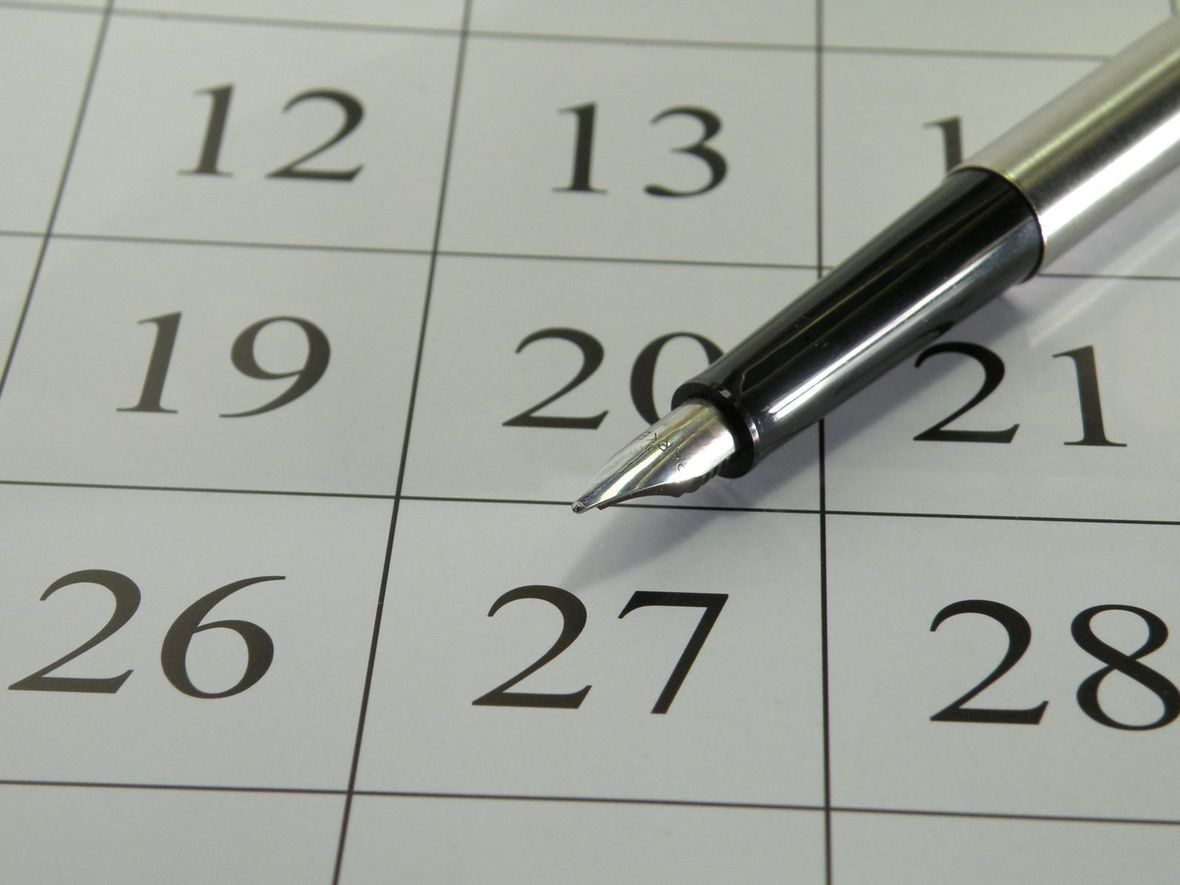# Music2yourfeet 7th Annual Holiday Party

 table div table+table+table+table+table+table+table+table+table+table+table+table+table+table+table+table+table+table+table div table{width:100%;padding:0}table div table+table+table+table+table+table+table+table+table+table+table+table+table+table+table+table+table+table+table div table img{width:96.23%;padding:0;float:none}table div table+table+table+table+table+table+table+table+table+table+table+table+table+table+table+table+table+table+table div table td{width:100%;padding:0 1.88% 18px}/* styles */## In the Spotlight.../* styles */ Congratulations to all the “2020 Graduates“all over the world. A special Congratulations to my great niece, Ashley Stinson, we are so proud of you. Congrats also to the Gardners, Stephanie, Stevenah, and Jake, all from the same family, that is Awesome!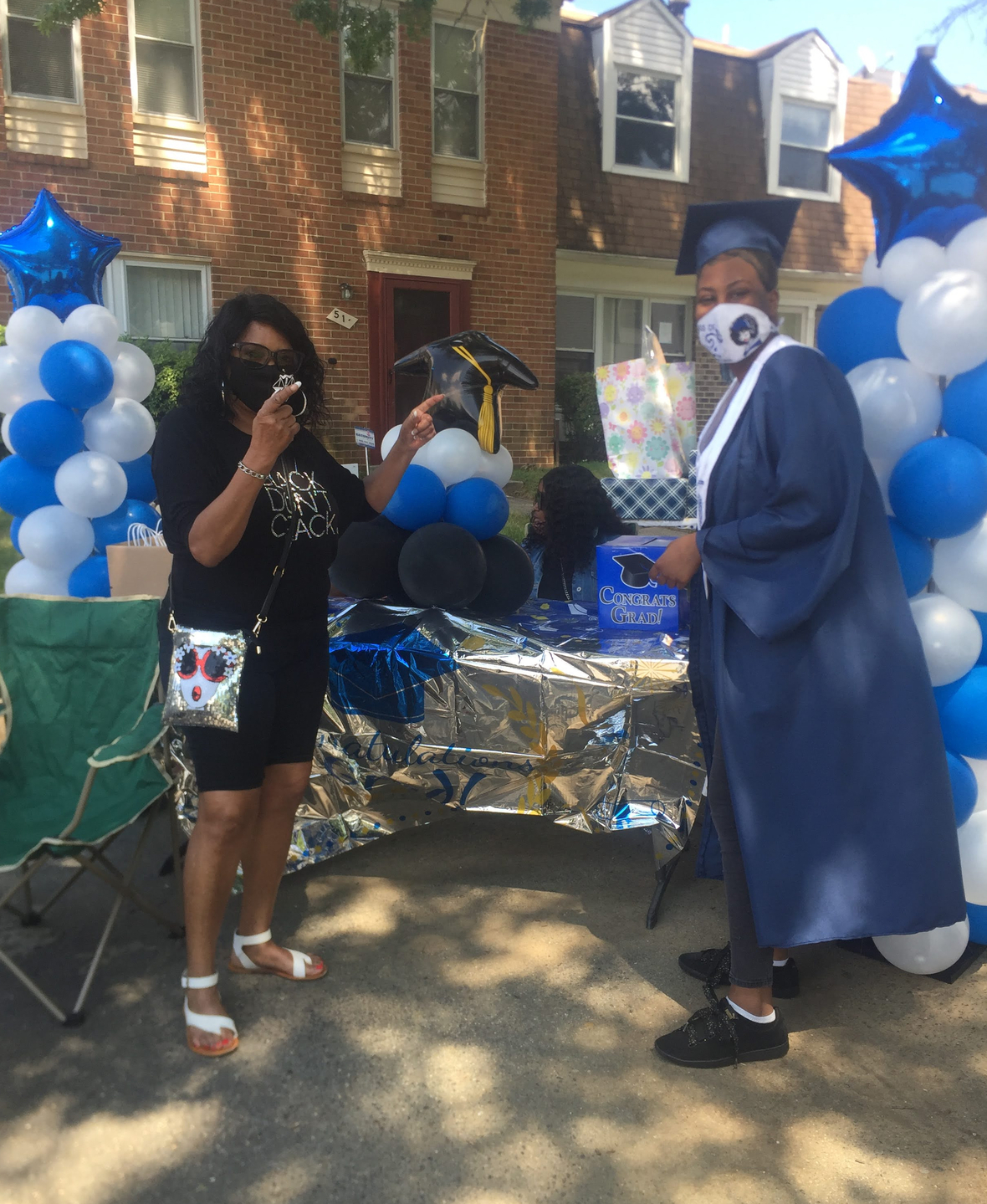/* styles */ Congratulations Xijala Veney, (Jayla), Central High School, Falcon Nation, class of 2020, Great job Jay, So proud of you, also Congratulations to her great aunt, Ann & great uncle, Walter Douglas, you are the best! She made it!
 table div table+table+table+table+table+table+table+table+table+table+table+table+table+table+table+table+table+table+table+table+table+table+table+table+table div table{width:100%;padding:0}table div table+table+table+table+table+table+table+table+table+table+table+table+table+table+table+table+table+table+table+table+table+table+table+table+table div table img{width:96.23%;padding:0;float:none}table div table+table+table+table+table+table+table+table+table+table+table+table+table+table+table+table+table+table+table+table+table+table+table+table+table div table td{width:100%;padding:0 1.88% 18px}/* styles */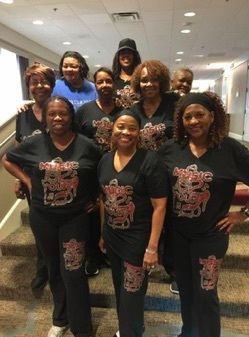Music2YourFeet Workout Suit (2 Piece)

\$40 for set, separate \$20 each,
come in black and gray only, stretch material...size from small to 2x pants and 3X shirt, may use Cash App \$seafo1 to pay, or cash, sorry no paypal and no checks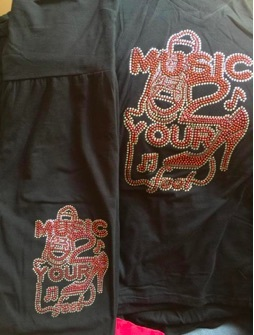Music2YourFeetWorkout Suit (pant and t-shirt)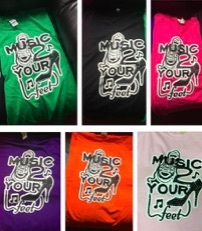Music2YourFeet T-Shirt

\$15 each, may use Cash App \$seafo1 to pay, or cash, sorry no paypal and no checks

Please indicate the T-shirt color when ordering.
Colors available:
Green, Black, Hot Pink, Purple, Orange and Light Pink,

 table div table+table+table+table+table+table+table+table+table+table+table+table+table+table+table+table+table+table+table+table+table+table+table+table+table+table+table+table+table+table div table{width:100%;padding:0}table div table+table+table+table+table+table+table+table+table+table+table+table+table+table+table+table+table+table+table+table+table+table+table+table+table+table+table+table+table+table div table img{width:96.23%;padding:0;float:none}table div table+table+table+table+table+table+table+table+table+table+table+table+table+table+table+table+table+table+table+table+table+table+table+table+table+table+table+table+table+table div table td{width:100%;padding:0 1.88% 18px}/* styles */## Special Birthday shout outs to my family and friends....Donnel Fletcher, Mike Middleton (Crazy Mike), Michael Richardson, and All the July babies...table div table+table+table+table+table+table+table+table+table+table+table+table+table+table+table+table+table+table+table+table+table+table+table+table+table+table+table+table+table+table+table+table+table+table div table{width:100%;padding:0}table div table+table+table+table+table+table+table+table+table+table+table+table+table+table+table+table+table+table+table+table+table+table+table+table+table+table+table+table+table+table+table+table+table+table div table img{width:96.23%;padding:0;float:none}table div table+table+table+table+table+table+table+table+table+table+table+table+table+table+table+table+table+table+table+table+table+table+table+table+table+table+table+table+table+table+table+table+table+table div table td{width:100%;padding:0 1.88% 18px}/* styles */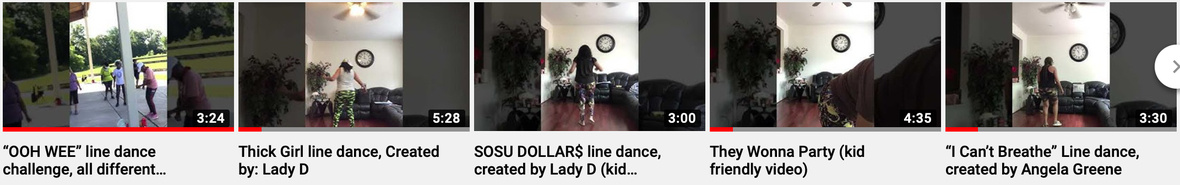www.music2yourfeet.com/videos.html
 /* styles */ View new line dance videos: http://www.music2yourfeet.com/videos.html
 /* styles */ Music2YourFeet visits many areas within the community, centers, parks and other events - spreading our love of line dancing. We capture every moment with video and photos. Please check out our photo gallery each month - you may find yourself in them - "Aroundtown"!
 table div table+table+table+table+table+table+table+table+table+table+table+table+table+table+table+table+table+table+table+table+table+table+table+table+table+table+table+table+table+table+table+table+table+table+table+table+table+table+table+table div table{width:100%;padding:0}table div table+table+table+table+table+table+table+table+table+table+table+table+table+table+table+table+table+table+table+table+table+table+table+table+table+table+table+table+table+table+table+table+table+table+table+table+table+table+table+table div table img{width:96.23%;padding:0;float:none}table div table+table+table+table+table+table+table+table+table+table+table+table+table+table+table+table+table+table+table+table+table+table+table+table+table+table+table+table+table+table+table+table+table+table+table+table+table+table+table+table div table td{width:100%;padding:0 1.88% 18px}/* styles *//* styles */ Special Thank you To front line employees who are helping to keep us safe, we love and appreciate you.## Interested in purchasing masks, and other items, here are just a few Folks to contact, all are on FB.

Andi Johnson, Lawanda & Sonya Gross, (L&S Wear),Velvet Johnson, (velvetprettybrowneyes Johnson), Angela Smith, (PA),Dees Master Crafts, Jaqui Bain (Memphis), Tiawana Silver, Tanya Dennis, (Bling Tees)

 table div table+table+table+table+table+table+table+table+table+table+table+table+table+table+table+table+table+table+table+table+table+table+table+table+table+table+table+table+table+table+table+table+table+table+table+table+table+table+table+table+table+table+table+table+table div table{width:100%;padding:0}table div table+table+table+table+table+table+table+table+table+table+table+table+table+table+table+table+table+table+table+table+table+table+table+table+table+table+table+table+table+table+table+table+table+table+table+table+table+table+table+table+table+table+table+table+table div table img{width:96.23%;padding:0;float:none}table div table+table+table+table+table+table+table+table+table+table+table+table+table+table+table+table+table+table+table+table+table+table+table+table+table+table+table+table+table+table+table+table+table+table+table+table+table+table+table+table+table+table+table+table+table div table td{width:100%;padding:0 1.88% 18px}/* styles */table div table+table+table+table+table+table+table+table+table+table+table+table+table+table+table+table+table+table+table+table+table+table+table+table+table+table+table+table+table+table+table+table+table+table+table+table+table+table+table+table+table+table+table+table+table+table+table div table td,table.module-46{width:100%;padding:0}table div table+table+table+table+table+table+table+table+table+table+table+table+table+table+table+table+table+table+table+table+table+table+table+table+table+table+table+table+table+table+table+table+table+table+table+table+table+table+table+table+table+table+table+table+table+table+table div table{width:100%;float:none;margin-left:auto;margin-right:auto;padding:0}table div table+table+table+table+table+table+table+table+table+table+table+table+table+table+table+table+table+table+table+table+table+table+table+table+table+table+table+table+table+table+table+table+table+table+table+table+table+table+table+table+table+table+table+table+table+table+table div table a{border:0 none;text-decoration:none}table div table+table+table+table+table+table+table+table+table+table+table+table+table+table+table+table+table+table+table+table+table+table+table+table+table+table+table+table+table+table+table+table+table+table+table+table+table+table+table+table+table+table+table+table+table+table+table div table img{width:100%!important;border:0 none;text-decoration:none}/* styles */
 table.module-47{width:75.47%;padding:0}table div table+table+table+table+table+table+table+table+table+table+table+table+table+table+table+table+table+table+table+table+table+table+table+table+table+table+table+table+table+table+table+table+table+table+table+table+table+table+table+table+table+table+table+table+table+table+table+table div table{width:75.47%;float:none;margin-left:auto;margin-right:auto;padding:0}table div table+table+table+table+table+table+table+table+table+table+table+table+table+table+table+table+table+table+table+table+table+table+table+table+table+table+table+table+table+table+table+table+table+table+table+table+table+table+table+table+table+table+table+table+table+table+table+table div table a{border:0 none;text-decoration:none}table div table+table+table+table+table+table+table+table+table+table+table+table+table+table+table+table+table+table+table+table+table+table+table+table+table+table+table+table+table+table+table+table+table+table+table+table+table+table+table+table+table+table+table+table+table+table+table+table div table img{width:100%!important;border:0 none;text-decoration:none}table div table+table+table+table+table+table+table+table+table+table+table+table+table+table+table+table+table+table+table+table+table+table+table+table+table+table+table+table+table+table+table+table+table+table+table+table+table+table+table+table+table+table+table+table+table+table+table+table div table td{width:100%;padding:0}/* styles */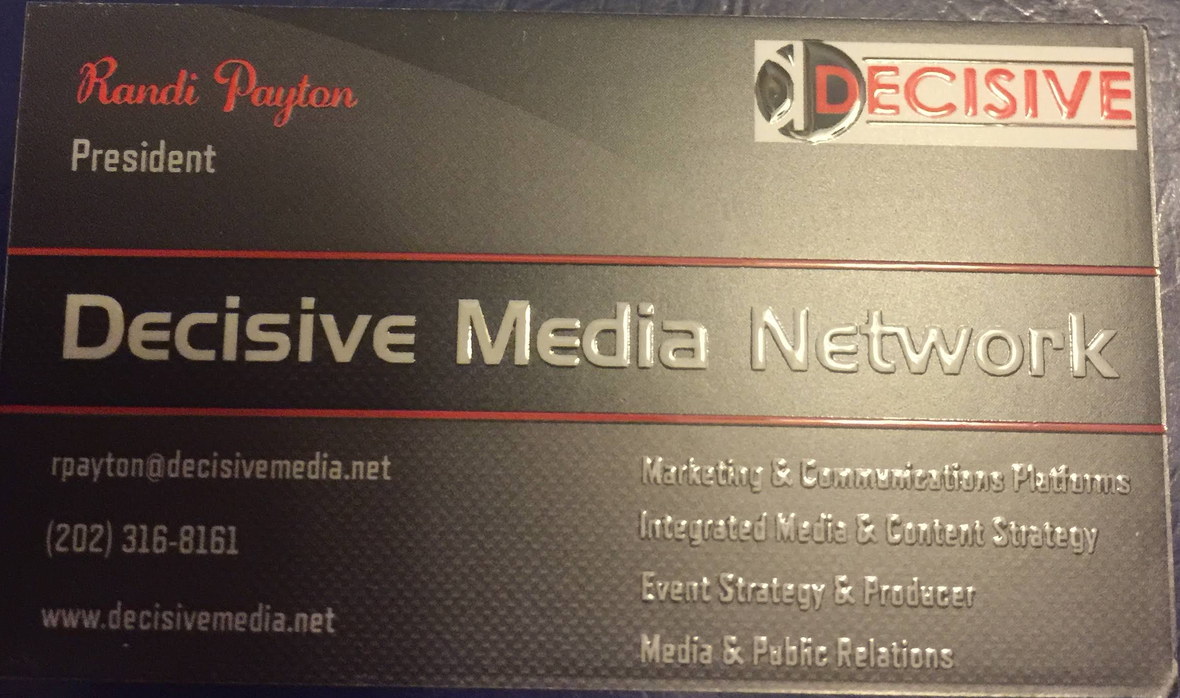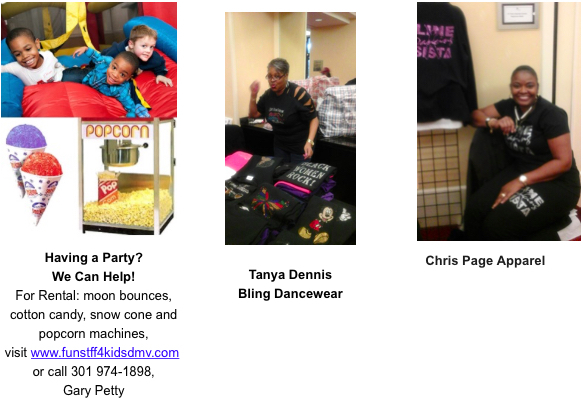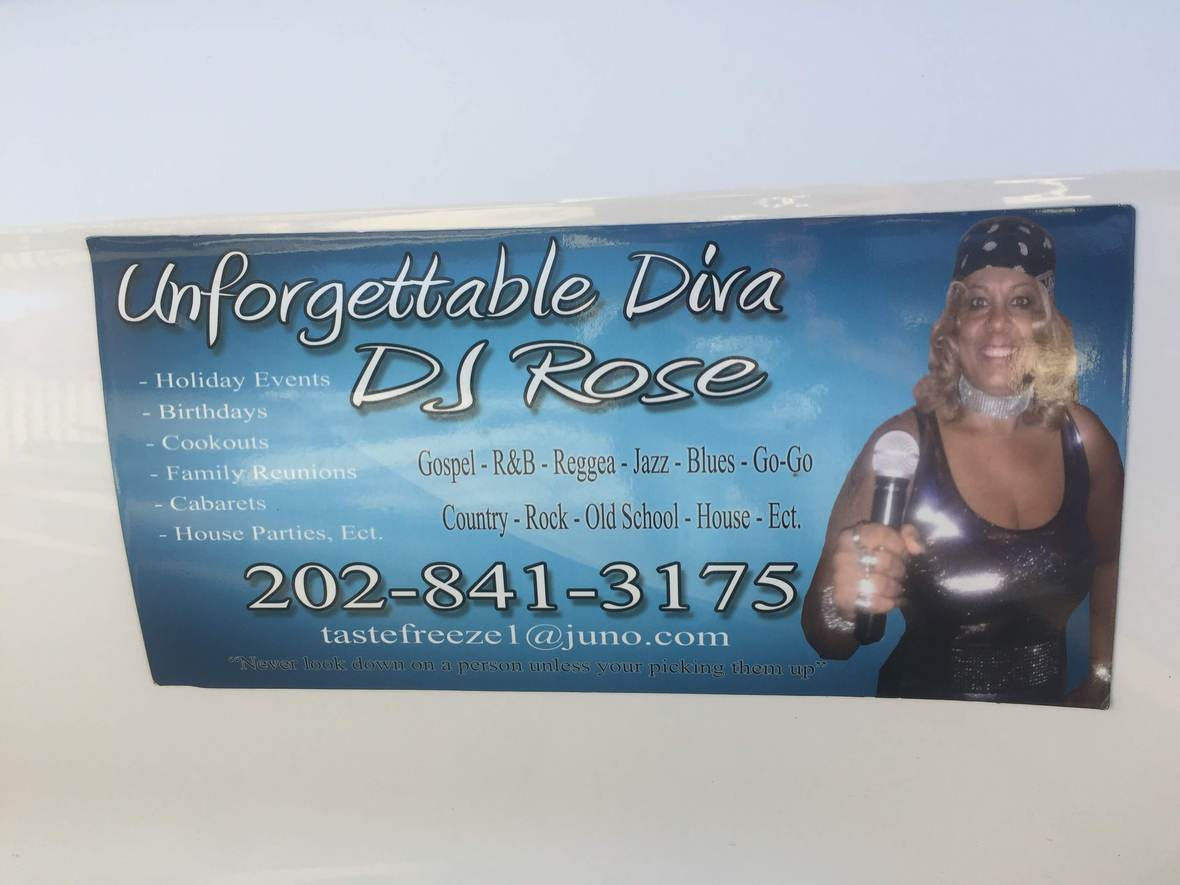# Thank you for your continued support!

 /* styles */ No word on classes at the recreation centers yet - will keep you informed.Classes and Social Events Cancelled Due to the Coronavirus Outbreak
As positive coronavirus cases have increased in Maryland and Gov. Larry Hogan (R) has declared a state of emergency, which has restricted public gatherings, businesses and government facilities, most public events have been canceled or postponed and public access to government services are increasingly restricted. With that all dance classes and social dance events are cancelled. Please check our website and Facebook page for updates.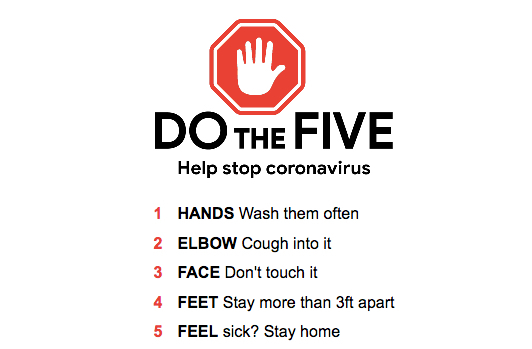table div table+table+table+table+table+table+table+table+table+table+table+table+table+table+table+table+table+table+table+table+table+table+table+table+table+table+table+table+table+table+table+table+table+table+table+table+table+table+table+table+table+table+table+table+table+table+table+table+table+table+table+table+table+table+table+table+table+table+table+table+table+table div table{width:100%;padding:0}table div table+table+table+table+table+table+table+table+table+table+table+table+table+table+table+table+table+table+table+table+table+table+table+table+table+table+table+table+table+table+table+table+table+table+table+table+table+table+table+table+table+table+table+table+table+table+table+table+table+table+table+table+table+table+table+table+table+table+table+table+table+table div table img{width:96.23%;padding:0;float:none}table div table+table+table+table+table+table+table+table+table+table+table+table+table+table+table+table+table+table+table+table+table+table+table+table+table+table+table+table+table+table+table+table+table+table+table+table+table+table+table+table+table+table+table+table+table+table+table+table+table+table+table+table+table+table+table+table+table+table+table+table+table+table div table td{width:100%;padding:0 1.88% 18px}/* styles */## Music2YourFeet Classes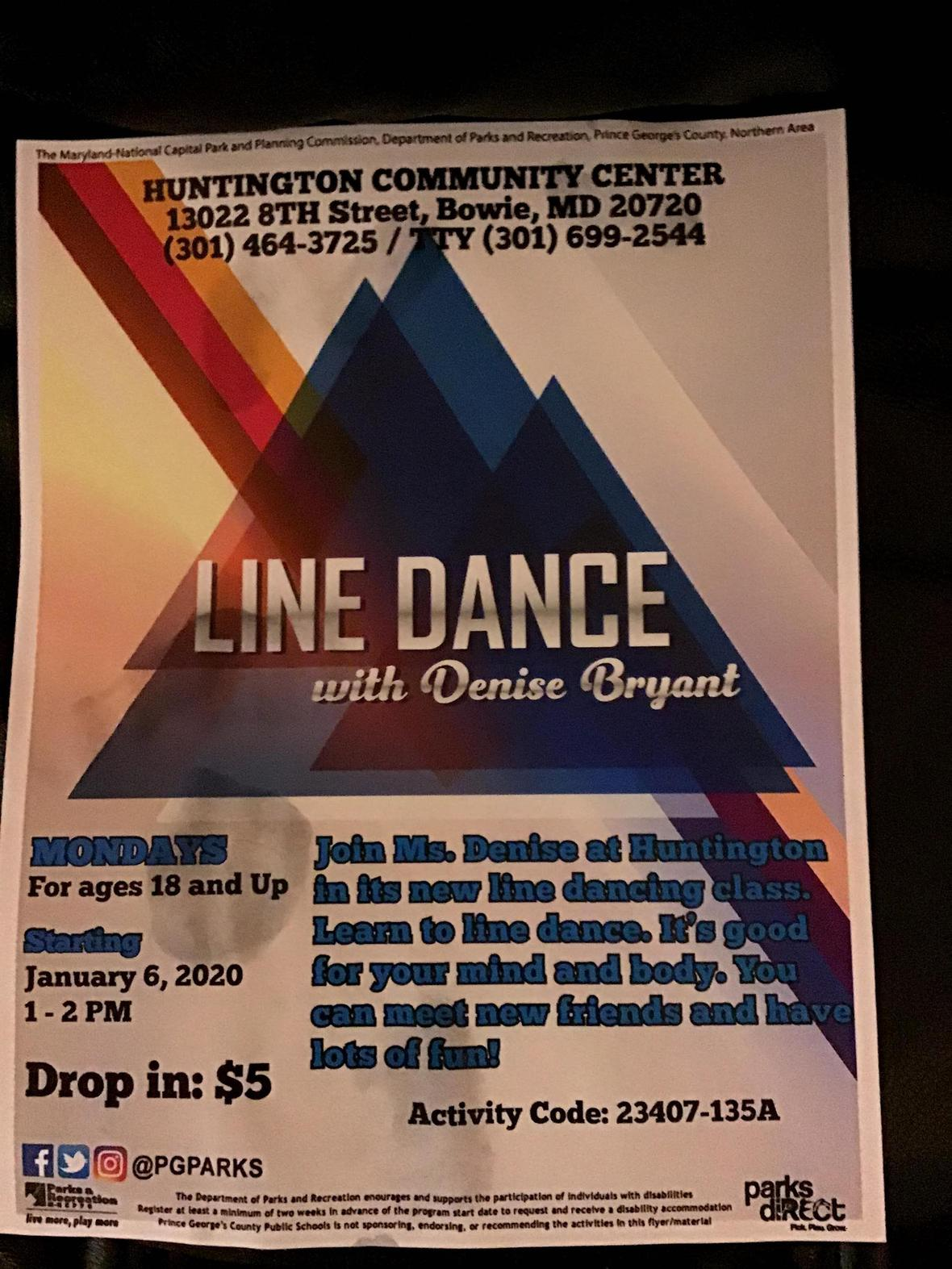Music2yourfeet line dance is at Huntington Community Center/Old Antique Bowie!

Beginners and Seniors, but all are welcome....come out for some fun and exercise, no experience necessary...every Monday at 1:00, see you there.

When: Mondays 1:00-2:00, \$5 drop in
Where: 13022 8th Street, Bowie, Md.

 /* styles */ Monday Lake Arbor Community Center 10100 Lake Arbor Way, Mitchellville, Md. 20721 10:00am-11:30am Calling all Beginners & Seniors, come out for some fun and exercise with Denise Monday Huntington Community Center 13022 8th Street Bowie, MD 1:00pm - 2:00pm \$5 drop in Tuesday Cedar Heights Community Center 1200 Glen Willow Drive Seat Pleasant, MD 9:00am-10:30am \$6, Intermediate Tuesday Glenn Dale Community Center 11901 Glenn Dale Blvd, Glenn Dale, MD 20769 11-12:30pm Beginner \$20 for residents and \$24 for Non-residents. \$5 drop in fee. Beginner Wednesday Good Luck Community Ctr. 6:30-8:00pm 8601 Good Luck Rd, Lanham, Md. 20706 Beginner Friday Ceder Heights Community Center 9am-10:30am 1200 Glen Willow Dr, Capitol Heights, MD 20743 Beginner Friday Senior Day Good Luck Community Center (on-going) 8601 Good Luck Rd Lanham, Md. 11:30am-1:30pm Seniors 60+ FREE, under 60 yrs. Old \$6 Music2YourFeet Private Lessons Available
 table div table+table+table+table+table+table+table+table+table+table+table+table+table+table+table+table+table+table+table+table+table+table+table+table+table+table+table+table+table+table+table+table+table+table+table+table+table+table+table+table+table+table+table+table+table+table+table+table+table+table+table+table+table+table+table+table+table+table+table+table+table+table+table+table+table+table+table div table{width:100%;padding:0}table div table+table+table+table+table+table+table+table+table+table+table+table+table+table+table+table+table+table+table+table+table+table+table+table+table+table+table+table+table+table+table+table+table+table+table+table+table+table+table+table+table+table+table+table+table+table+table+table+table+table+table+table+table+table+table+table+table+table+table+table+table+table+table+table+table+table+table div table img{width:96.23%;padding:0;float:none}table div table+table+table+table+table+table+table+table+table+table+table+table+table+table+table+table+table+table+table+table+table+table+table+table+table+table+table+table+table+table+table+table+table+table+table+table+table+table+table+table+table+table+table+table+table+table+table+table+table+table+table+table+table+table+table+table+table+table+table+table+table+table+table+table+table+table+table div table td{width:100%;padding:0 1.88% 18px}/* styles */## DMV Monthly Dance Socials

 /* styles */ First Sunday of Each Month Sunday Line Dance Social - at Cedar Heights Community Center, 1200 Glen Willow Drive, Seat Pleasant, Md. 3pm - 7pm \$6 for Res & Non-Res. First Friday Line Dance Socials with April Love Jones Southern Regional Technology and Recreation Complex, 6pm-9:30pm includes light refreshments, \$5 resident \$6 non resident First and Third Friday Delight featuring DJ Zone @ VFW Post 7916 (upstairs), 204 Mill Street Occoquan, Va 22125. Time: 7:30 PM to 11:30 PM. Cost: \$10. Open dancing, Cash Bar, Bring your own Food or purchase from the VFW. Follow DJ Zone on Facebook under Vincent Price and join my DJ Zone Status Group for other events and dates. First Saturday of Each Month - Toast it Up Productions - Shut Up & Dance, 1:00-5:00pm, Elk's Lodge, 7350 Temple Hills, Md. BYOF, \$7.00 Third Saturday of Each Month S.O.S. (Seniors On Step) line dance social; 6:00-10:00pm; Cost: Free; BYOFood & beverage (no alcohol permitted) Kingstowne Community Center for Active Adults, 6488 Landsdowne Centre Drive, Alexandria, VA 22315. Every Second Friday, \$5 6p-9p. Learn the latest line dances with Peytrienne! All are welcome, water provided, Soul Line & Hand dance Party, White Oak Community Center, 1700 April Lane Silver Spring, Md. 20904 Every Sunday Elks Lodge of Camp Springs 7350 Temple Hills Rd Temple Hills Md, Hand Dance, Bop, Line Dance & more 6p-10p Fourth Friday of the Month - Style & Rhythm, 4th Friday social ,\$5, Ridgley's Run Community Ctr. 8400 Mission Road, Jessup, Md.
 table div table+table+table+table+table+table+table+table+table+table+table+table+table+table+table+table+table+table+table+table+table+table+table+table+table+table+table+table+table+table+table+table+table+table+table+table+table+table+table+table+table+table+table+table+table+table+table+table+table+table+table+table+table+table+table+table+table+table+table+table+table+table+table+table+table+table+table+table+table+table div table{width:100%;padding:0}table div table+table+table+table+table+table+table+table+table+table+table+table+table+table+table+table+table+table+table+table+table+table+table+table+table+table+table+table+table+table+table+table+table+table+table+table+table+table+table+table+table+table+table+table+table+table+table+table+table+table+table+table+table+table+table+table+table+table+table+table+table+table+table+table+table+table+table+table+table+table div table img{width:96.23%;padding:0;float:none}table div table+table+table+table+table+table+table+table+table+table+table+table+table+table+table+table+table+table+table+table+table+table+table+table+table+table+table+table+table+table+table+table+table+table+table+table+table+table+table+table+table+table+table+table+table+table+table+table+table+table+table+table+table+table+table+table+table+table+table+table+table+table+table+table+table+table+table+table+table+table div table td{width:100%;padding:0 1.88% 18px}/* styles *//* styles */ Denise's "Music2YourFeet" Line Dancing provides you with the opportunity to exercise socialize and have fun - all in one setting. Break the monotony of a long day at work, (evening classes); get mid-day relief to an otherwise stressful day (lunch time classes); Relax after a long work week (weekend classes); or one-on-one personal service (private classes, no matter the day, it will be music2yourfeet Submit your events. Donations not required but appreciated.
 table div table+table+table+table+table+table+table+table+table+table+table+table+table+table+table+table+table+table+table+table+table+table+table+table+table+table+table+table+table+table+table+table+table+table+table+table+table+table+table+table+table+table+table+table+table+table+table+table+table+table+table+table+table+table+table+table+table+table+table+table+table+table+table+table+table+table+table+table+table+table+table+table+table div table{width:100%;padding:0}table div table+table+table+table+table+table+table+table+table+table+table+table+table+table+table+table+table+table+table+table+table+table+table+table+table+table+table+table+table+table+table+table+table+table+table+table+table+table+table+table+table+table+table+table+table+table+table+table+table+table+table+table+table+table+table+table+table+table+table+table+table+table+table+table+table+table+table+table+table+table+table+table+table div table img{width:96.23%;padding:0;float:none}table div table+table+table+table+table+table+table+table+table+table+table+table+table+table+table+table+table+table+table+table+table+table+table+table+table+table+table+table+table+table+table+table+table+table+table+table+table+table+table+table+table+table+table+table+table+table+table+table+table+table+table+table+table+table+table+table+table+table+table+table+table+table+table+table+table+table+table+table+table+table+table+table+table div table td{width:100%;padding:0 1.88% 18px}/* styles */## More Line Dance Classes

 /* styles */ Garland Christopher Tuesday only class 11:00a-12:00pm Palmer Park Community Center 7720 Barlowe Rd, Landover, MD 20785 (301) 773-5665
 table div table+table+table+table+table+table+table+table+table+table+table+table+table+table+table+table+table+table+table+table+table+table+table+table+table+table+table+table+table+table+table+table+table+table+table+table+table+table+table+table+table+table+table+table+table+table+table+table+table+table+table+table+table+table+table+table+table+table+table+table+table+table+table+table+table+table+table+table+table+table+table+table+table+table+table+table div table{width:100%;padding:0}table div table+table+table+table+table+table+table+table+table+table+table+table+table+table+table+table+table+table+table+table+table+table+table+table+table+table+table+table+table+table+table+table+table+table+table+table+table+table+table+table+table+table+table+table+table+table+table+table+table+table+table+table+table+table+table+table+table+table+table+table+table+table+table+table+table+table+table+table+table+table+table+table+table+table+table+table div table img{width:96.23%;padding:0;float:none}table div table+table+table+table+table+table+table+table+table+table+table+table+table+table+table+table+table+table+table+table+table+table+table+table+table+table+table+table+table+table+table+table+table+table+table+table+table+table+table+table+table+table+table+table+table+table+table+table+table+table+table+table+table+table+table+table+table+table+table+table+table+table+table+table+table+table+table+table+table+table+table+table+table+table+table+table div table td{width:100%;padding:0 1.88% 18px}/* styles */## Upcoming Events

 /* styles */ Classes and Social Events Cancelled Due to the Coronavirus Outbreak As positive coronavirus cases have increased in Maryland and Gov. Larry Hogan (R) has declared a state of emergency, which has restricted public gatherings, businesses and government facilities, most public events have been canceled or postponed and public access to government services are increasingly restricted. With that all dance classes and social dance events are cancelled. Please check our website and Facebook page for updates.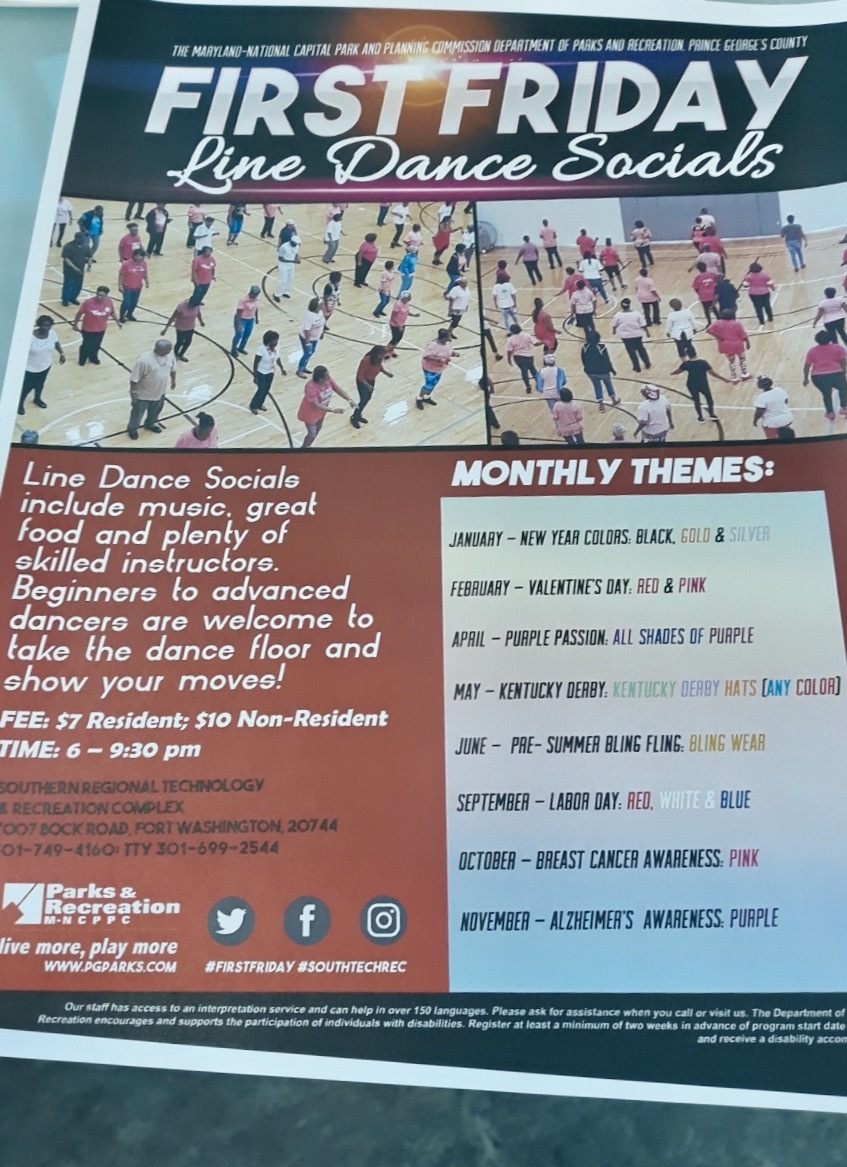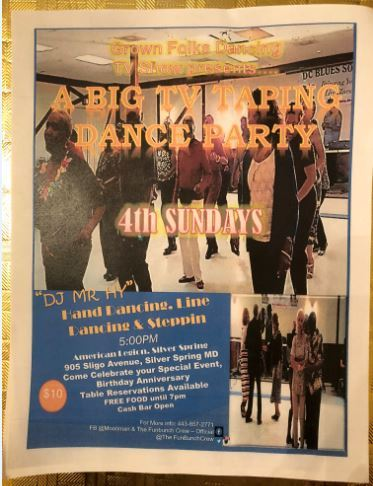table div table+table+table+table+table+table+table+table+table+table+table+table+table+table+table+table+table+table+table+table+table+table+table+table+table+table+table+table+table+table+table+table+table+table+table+table+table+table+table+table+table+table+table+table+table+table+table+table+table+table+table+table+table+table+table+table+table+table+table+table+table+table+table+table+table+table+table+table+table+table+table+table+table+table+table+table+table+table+table+table+table+table div table{width:100%;padding:0}table div table+table+table+table+table+table+table+table+table+table+table+table+table+table+table+table+table+table+table+table+table+table+table+table+table+table+table+table+table+table+table+table+table+table+table+table+table+table+table+table+table+table+table+table+table+table+table+table+table+table+table+table+table+table+table+table+table+table+table+table+table+table+table+table+table+table+table+table+table+table+table+table+table+table+table+table+table+table+table+table+table+table div table img{width:96.23%;padding:0;float:none}table div table+table+table+table+table+table+table+table+table+table+table+table+table+table+table+table+table+table+table+table+table+table+table+table+table+table+table+table+table+table+table+table+table+table+table+table+table+table+table+table+table+table+table+table+table+table+table+table+table+table+table+table+table+table+table+table+table+table+table+table+table+table+table+table+table+table+table+table+table+table+table+table+table+table+table+table+table+table+table+table+table+table div table td{width:100%;padding:0 1.88% 18px}/* styles */